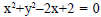The equationrepresents
• a)
circle
• b)
ellipse
• c)
pair of lines
• d)
no real curve

### Related TestDivya Patel Dec 05, 2021
-by this equation it seems circle with center (-1,0)
but its not a real circle because radius =
√(g^2+f^2-c)=√(-1)
so it's not a circle
-for ellipse a>b but here both are same so not ellipse.
-for pair of straight lines take diterminant
|a h g|. put the value in this diterminant
|h b f|. if the answer of this is 0 so it's a
|g f c|. straight line. but on solving this we are getting a number so it's not a straight line
so option d is correct

-by this equation it seems circle with center (-1,0)but its not a real circle because radius =√(g^2+f^2-c)=√(-1)so it's not a circle-for ellipse a>b but here both are same so not ellipse.-for pair of straight lines take diterminant |a h g|. put the value in this diterminant |h b f|. if the answer of this is 0 so it's a |g f c|. straight line. but on solving this we are getting a number so it's not a straight line so option d is correct
-by this equation it seems circle with center (-1,0)but its not a real circle because radius =√(g^2+f^2-c)=√(-1)so it's not a circle-for ellipse a>b but here both are same so not ellipse.-for pair of straight lines take diterminant |a h g|. put the value in this diterminant |h b f|. if the answer of this is 0 so it's a |g f c|. straight line. but on solving this we are getting a number so it's not a straight line so option d is correct